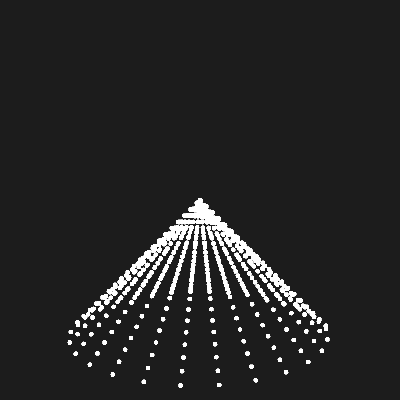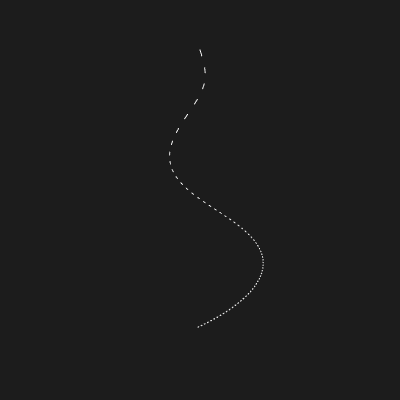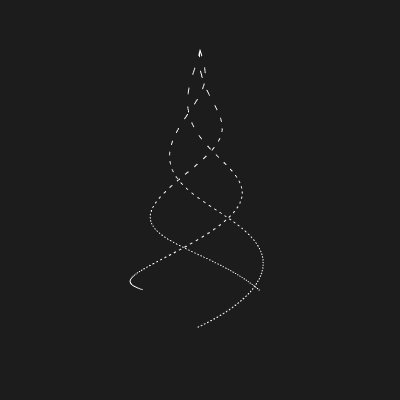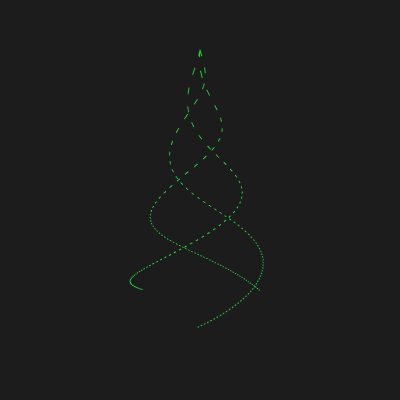# Christmas Tree

Merry Christmas!

Yesterday, I came across an animated Christmas tree. I wanted to reproduce this myself.

The basic idea is that this each “line” on the tree is just a spiral. And you draw a spiral by drawing a circle with an ever-increasing radius.

Normally, you draw a circle with `x(t) = cos(t)` and `y(t) = sin(t)`. The approach here increases the radius by scaling against `t`: `x(t) = t * cos(t)` and `y(t) = t * sin(t)`. The third dimension is just `t`.

This actually draws the tree with the base towards you, so we rotate it:

``````x(t) = t * cos(t)
y(t) = t
z(t) = t * sin(t)
``````

Trying it out:

``````function draw() {
stroke(255);
let t = frameCount;
point(cos(t) * t, sin(t) * t, t);
}
``````

Looks nice, but not what I was expecting:Of course, the issue was that I forgot to rotate it.

``````stroke(255);
let t = frameCount * TWO_PI / 30;
point(cos(t) * t, t, sin(t) * t);

if (frameCount == 600) {
noLoop();
}
``````This is good! A lot of tweaks later, I had a nice curve:There was quite a bit that had to be updated to get to this point. I translated the starting point, changed the stroke weight, and messed around a lot with scaling constants.

Most important, I changed the step increment unit from angles to arc length. The process for this is described here. Then, instead of increasing the angle by a constant amount, you increase the arc length by a constant amount.

This is supposed to make the points uniformly spaced. This should be correct in my image, but visually it looks further at the top. I’m assuming this is because of small, pixel distances (and because I don’t know how to manipulate the camera).

It gets better once we draw a line partway between each point:Finally, we throw in some additional spirals, each with some rotational offset!Time-based rotation and some color gives us our final tree!Sketch available here.